# LEN Function Excel - A Beginner's GuideClaudia Buckley#### Join the Excel conversation on Slack

Ask a question or join the conversation for all things Excel on our Slack channel.

The LEN Excel function returns the number of characters in a text string. It is also known as the length function in Excel, and can be found among the text category of formulas.

Use this free LEN Excel file to practice along with the tutorial.

## Syntax - LEN function Excel

The LEN function carries only one argument, which is the text it is being asked to evaluate.

``=LEN(text)``

That text may be in the form of a cell reference, or text typed directly into the formula within double quotation marks.

Interestingly, LEN will also count the number of characters in numeric values, but it counts the number of characters in the true value of the number, and not necessarily the format which is displayed.

Therefore, in the following image, the number in cell A2 is counted as 3 characters, since the true value is 100, although the number is formatted with 7 characters.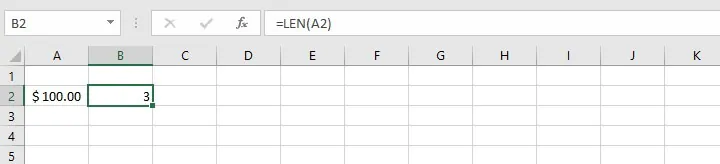Conversely, in the following image, the number in cell A2 is counted as 17 characters although the decimal was rounded to 6 places and the cell only displays 8 characters.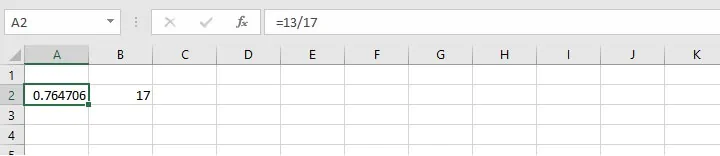If you only wanted to learn what the LEN function does, you just did. But you probably also want to know how you can apply it to solve problems or use it to improve your workflow. Here are a few examples.

## Combine with other string functions

LEN is often combined with other Excel string functions like TRIM, LEFT, MID, RIGHT, and SEARCH to extract specific elements from a longer text string. It’s important to know the type of value returned for each function in order to determine the right combination. A summary of the common text functions in this category are shown below.

Function

Syntax

Return value

LEN

LEN(text)

Number of characters

TRIM

TRIM(text)

Text without leading and trailing spaces

SUBSTITUTE

SUBSTITUTE(text, old_text, new_text, [instance_num])

Text, by substituting given values

LEFT

LEFT(text, [num_chars])

Text - leftmost character(s)

RIGHT

RIGHT (text, [num_chars])

Text - rightmost character(s)

MID

MID(text, start_num, num_chars)

Text - middle characters, given left start and length

SEARCH

SEARCH(find_text, within_text, [start_num])

Position number of specified text

### Exclude leading and trailing spaces with TRIM

In the example below, the LEN function in cell B1 is set to count the number of characters in cell A1 and returns a value of 20.

``=LEN(A1)``This seems incorrect until we notice that the phrase “Happy anniversary!” in A1 has a space before and after the text, so instead of getting a count of 18 characters, the LEN function counted a total of 20.

To eliminate the possibility of this happening (which occurs fairly often, especially with imported text), we can nest the TRIM and LEN functions. TRIM is designed to remove all spaces from a text string except for the spaces between words, and display the remaining characters. Placing the TRIM formula as the argument of the LEN function tells Excel to count the number of characters in the trimmed string.

``=LEN(TRIM(A1))``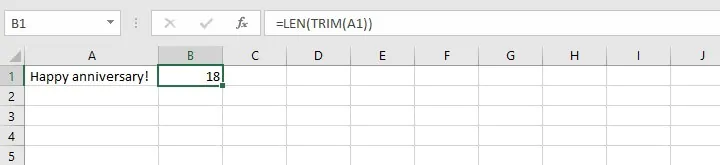Note that the space between “happy” and “anniversary” as well as the exclamation mark are all counted.

### Exclude all spaces

We can also use the LEN function to count the characters in a cell, excluding all spaces, even the ones between words. To achieve this, we can use the SUBSTITUTE and LEN formula combination.

The syntax of the SUBSTITUTE function is:

``=SUBSTITUTE(text, old_text, new_text, [instance_num])``

The text to be replaced (old text) and replacement text (new text) are each placed in double quotes. The instance_num argument specifies which occurrence of the old text should be replaced. Since we want all spaces to be replaced, we will omit this optional argument.

Nesting the two functions gives the formula:

``=LEN(SUBSTITUTE(A1," ",""))``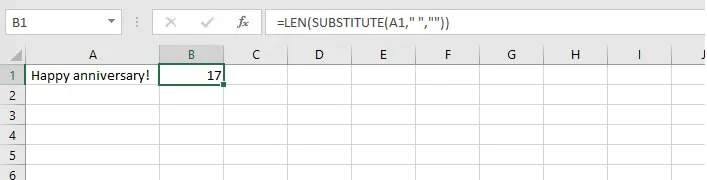Note that a space is placed between two double quotation marks for the old_text argument, and no characters are placed between double quotes for the new_text argument.

## Counting strings of variable length

LEN can also be combined with various functions to count the number of characters in a specific portion of a text string, and is especially useful when text follows a particular format but can be of variable length. The main thing to bear in mind is the type of data each function returns and how we can incorporate that into a useful formula.

### Using LEN with character SEARCH

For example, the product codes below follow a pattern, but may have either two or three numbers to the right and left of each dash.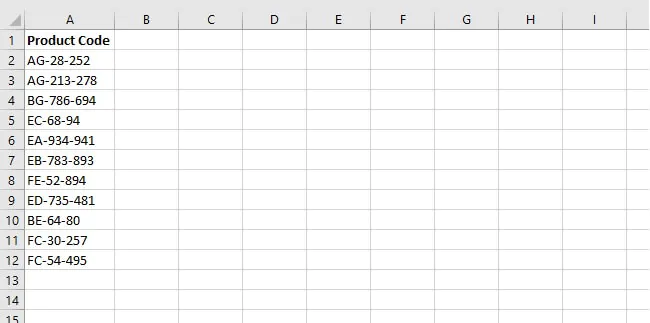It’s possible that the number of characters has some significance and we want to identify which product codes have three numbers in the last group. We can think about what we are asking in everyday terms — “How many characters appear after the second dash?”

One suggestion when using Excel to extract text from a cell after a character is to use any type of consistency to your advantage. We know that with the above product codes, the first dash will always be the third character, so we are really interested in the fourth and subsequent characters. We can do a search to find out the position of the second dash with the SEARCH function.

``=SEARCH("-",A2,4)``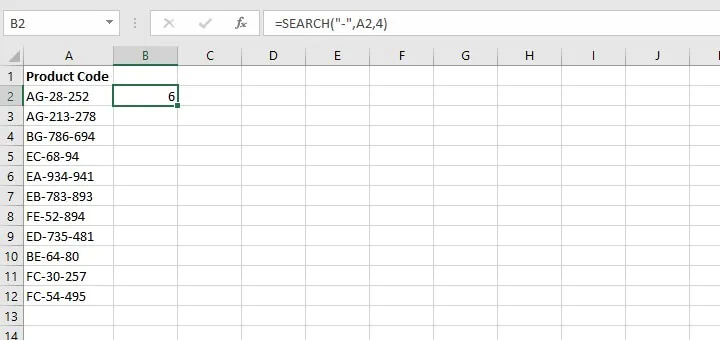This formula tells us that the second dash is the sixth character in the first product code (the entire text string AG-28-252). Now we just need to determine the length of the product code by using the LEN function, then we can subtract the result of the above formula.

``=LEN(A2) - SEARCH("-",A2,4)``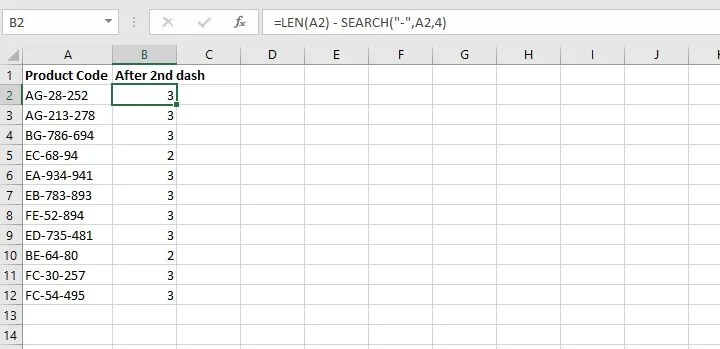Extracting and counting the number of characters in the middle of this string is trickier, especially because two of the three groups of characters are of variable lengths, but it can be done. The LEFT, RIGHT, and MID functions are often used to truncate text in Excel.

### Using LEN with the MID function

Remember, we want to use the consistency of the first three characters to our advantage. The MID function requires the starting point, which we know will be the fourth number, but it also needs to know how many characters the “middle string” consists of. Well, we don’t know that, and it is in fact exactly the value we are trying to find out.

Hot tip: Fortunately, we can treat the entire string after the dash as our middle string by specifying a number that is very large. For the sake of this example, we will use 100.

``=MID(A2,4,100)``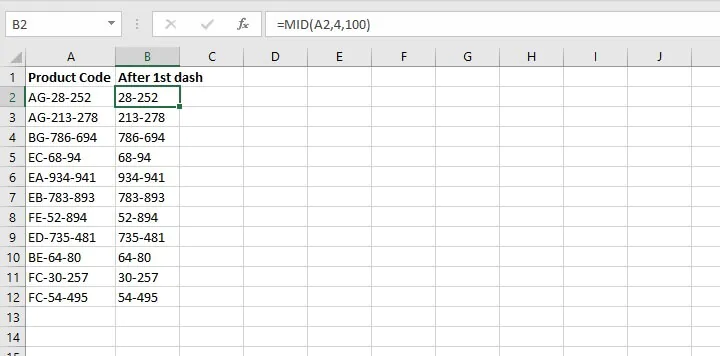• The MID function returns the truncated text specified by the formula — in the example above, beginning with the text after the fourth character (right after the first dash) in cell A2.
• An extreme value of 100 is used as the last argument in the MID formula to ensure that all characters which appear after the first dash are returned in cell B2.

Now all we need to do is grab the characters before the dash in column B and have the LEN function count them.

``=LEN(LEFT(B2,SEARCH("-",B2)-1))``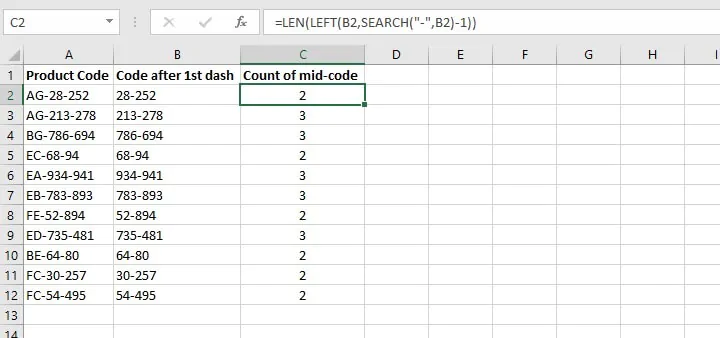Let’s break down what each portion of the above-nested formula does.

• ``SEARCH("-",B2)-1``

This searches in cell B2 for a dash, returns the position number and subtracts the number 1. For the value 28-252 the dash is in position 3. Subtract 1 and we have a value of 2.

• ``LEFT(B3, SEARCH("-",B2)-1)``

This uses the result of the SEARCH formula to get the second argument, num_chars. The LEFT function looks at the text in cell B2 and returns the 2 leftmost characters, 28.

• ``LEN(LEFT(B2,SEARCH("-",B2)-1))``

This counts the number of characters in the result of the above formula, 28. There are 2 characters, which provides the answer to our original question.

## LEN vs LENB

If you’re interested in learning about LENB, you’ll want to know that the only difference between LEN and LENB is that LEN returns the number of characters in a text string, while LENB returns the number of bytes used to represent the characters in a text string.

LENB counts 2 bytes per character only when a double-byte character set (DBCS) language is set as the default language. The languages that support DBCS include Japanese, Chinese (Simplified), Chinese (Traditional), and Korean.

Otherwise, LENB behaves the same as LEN, counting 1 byte per character. For this reason, we have only discussed LEN in this resource.

## Conclusion - LEN function Excel

LEN is the simple formula that can solve complex problems. Maybe there are other ways you’ve found LEN to be helpful. Let us know in the comments, and get more useful tips from our other resource articles.

You can also try our Excel - Basic and Advanced course to learn about other useful Excel tools or start with our free Excel in an Hour course to cover some basics in Excel.

Free Excel crash course

Learn Excel essentials fast with this FREE course. Get your certificate today!#### Join the Excel conversation on Slack

Ask a question or join the conversation for all things Excel on our Slack channel.Claudia Buckley

Claudia is a project manager and business skills instructor at GoSkills. In her spare time, she reads mystery novels and does genealogy research.